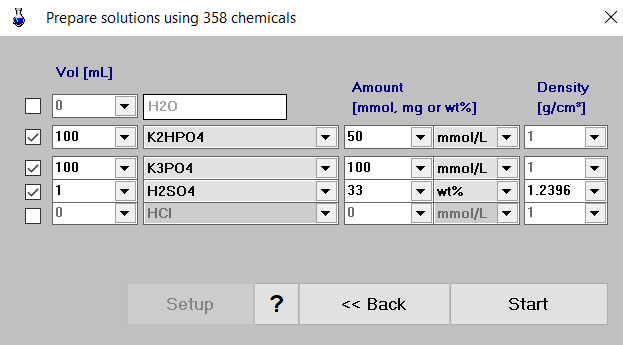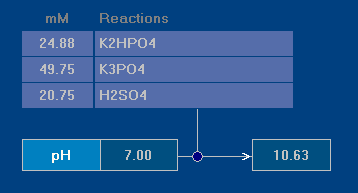# Preparation of Solutions and Buffers

Aqueous solutions and buffers can be prepared by mixing of up to four chemicals or substances (plus H2O) at 25°C. The amount of each chemical is entered in one of the following ways:

 mmol number of millimoles in a specified volume mmol/L molar concentration mg mass of chemical in a specified volume mg/L mass concentration wt% weight percent (mass percentage w/w)

The latter case (input as wt%) requires the density (specific gravity) of the corresponding aqueous solution as an additional parameter. For this purpose aqion provides the density of about 60 inorganic and organic solutions interpolated from Perry’s Chemical Engineers’ Handbook.1

ExampleWhat is the pH of a solution that consists of three components:

• 100 mL of 0.05 M K2HPO4
• 100 mL of 0.10 M K3PO4
• 1 mL of 33% (w/w) H2SO4 ?

The corresponding input panel is shown in the right screenshot. Please note that for H2SO4, and only for this item, a density value is required (here 1.2396 g/cm3 as provided by table interpolation1).

The calculation is performed in two steps. First, all components are converted to chemical units mmol/L (= millimoles per total volume of the final solution) which then enter the equilibrium calculation (second step).Results. The final solution obeys an pH value of

pH = 10.71

as shown in the right screenshot.

References

1. Perry’s Chemical Engineers’ Handbook 7th Edition by Perry, Robert H.; Green, Don W., McGraw-Hill, 1997  2